• 在既往文章中，我们已经介绍了怎么使用R语言可视化visreg包对交互作用进行可视化分析（见下图）， 但是使用visreg包后我们对图形的细节掌控力不够，今天我们使用重新绘制手动图形，这在SCI论文中非常实用，几乎可以...
交互作用效应(p for Interaction)在SCI文章中可以算是一个必杀技，几乎在高分的SCI中必出现，因为把人群分为亚组后再进行统计可以增强文章结果的可靠性，不仅如此，交互作用还可以使用来进行数据挖掘。在既往文章中，我们已经介绍了怎么使用R语言可视化visreg包对交互作用进行可视化分析（见下图），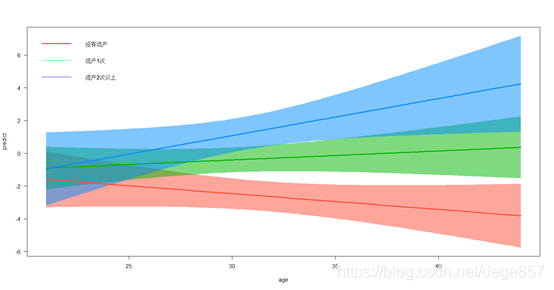但是使用visreg包后我们对图形的细节掌控力不够，今天我们使用重新绘制手动图形，这在SCI论文中非常实用，几乎可以做出所有的交互可视化，也有利于我们了解制图原理。 废话不多说，我们实操一下。我们使用人流后导致不孕的数据集(关注公众号后回复：不孕症，可以获得数据)，我们先导入看一下
library(rms)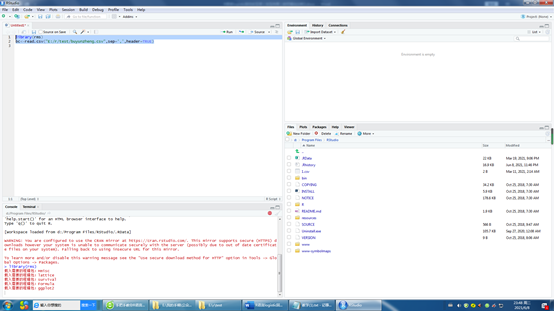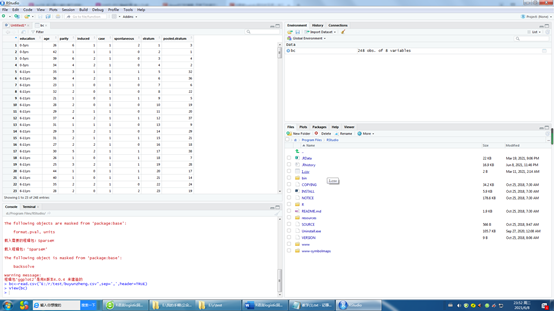数据有8个指标，最后两个是PSM匹配结果，我们不用理他，其余六个为： Education：教育程度，age:年龄，parity产次，induced：人流次数，case：是否不孕，这是结局指标，spontaneous：自然流产次数。 有一些变量是分类变量，我们需要把它转换一下
bc$education<-ifelse(bc$education=="0-5yrs",0,ifelse(bc$education=="6-11yrs",1,2)) bc$spontaneous<-as.factor(bc$spontaneous) bc$case<-as.factor(bc$case) bc$induced<-as.factor(bc$induced) bc$education<-as.factor(bc$education)  建立模型，假如我们想知道流产（包括人流和自然流产）和年龄这两个指标是不是存在交互影响 f1<- glm(case ~ age + education + parity + induced+spontaneous+age*induced*spontaneous, family = binomial(link = logit), data = bc) summary(f1)  我们看到自然流产和年龄之间可能存在交互（下图）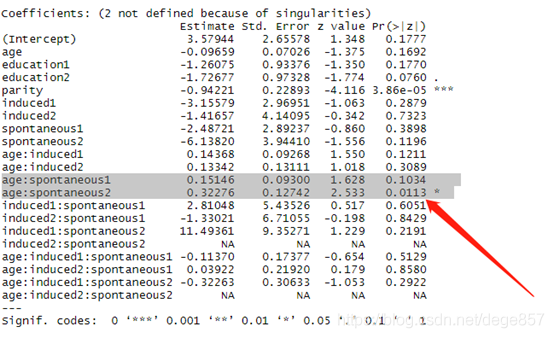重新建立模型 f1<- glm(case ~ age + parity +spontaneous+age:spontaneous, family = binomial(link = logit), data = bc)  建立新数据集 attach(bc) newdata2<-data.frame(age,spontaneous,parity=mean(parity))  把模型带入新数据集 newdata3<-data.frame(newdata2,predict(f1,newdata = newdata2,type = "link",se =T))  生成预测值 newdata4<-within(newdata3,{ pre<-plogis(fit) ll<-plogis(fit-(1.96*se.fit)) ul<-plogis(fit+(1.96*se.fit)) })  最后绘图 ggplot(newdata4,aes(x=age,y=pre))+ geom_line(aes(col=spontaneous),size=1)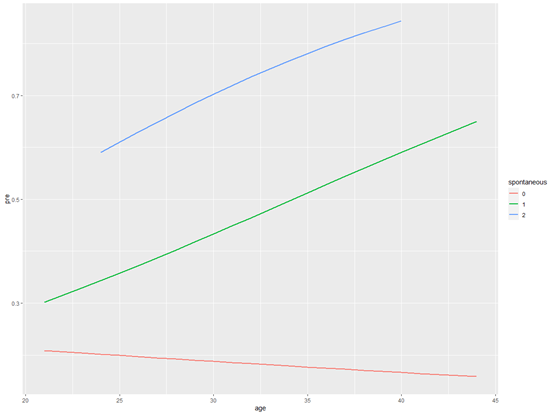最后对图形进行修饰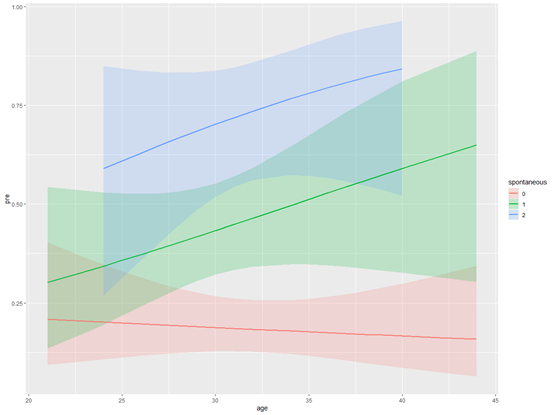最后得出和visreg包同样的结果，随着年龄增长，自然流产2次以上的患者不孕的概率比没有自然流产的患者明显增高。 我们还可以在细节和年龄范围进一步修饰，这里就不多说了，总之，数据交互可视化是一项非常实用的技能，不仅可以用于数据分析，还可以用于数据挖掘。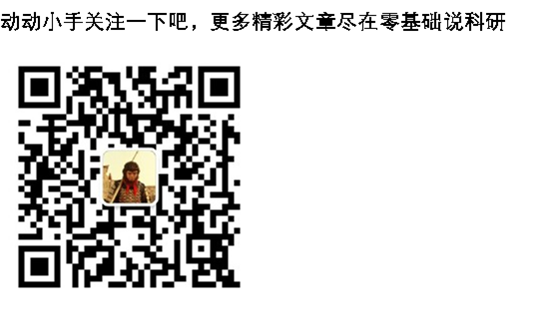展开全文数据分析 数据挖掘 • 最近做数据分析时，入坑了R语言，画了一些感觉很有趣的交互图，现在把它分享出来，方便大家参考，毕竟独乐乐不如众乐乐。在此做个记录，也方便日后自己查找！1.云图-----显示的是我本地数据库所有新闻共同提到的热点...  最近做数据分析时，入坑了R语言，画了一些感觉很有趣的交互图，现在把它分享出来，方便大家参考，毕竟独乐乐不如众乐乐。在此做个记录，也方便日后自己查找！ 1.云图-----显示的是我本地数据库所有新闻共同提到的热点词汇 (注：需要数据分析与挖掘的部分知识，可以参考我之前写的文章)R代码部分： library(wordcloud2) library(stringr) library(plyr) f words for(i in 1:length(f)) { words[i] } words words tableWord tableWord = tableWord[c(16:4000),] letterCloud(tableWord,word="LCB",size = 10) 2.饼图----展示的是各大城市职位的组成代码部分： library(RODBC) par(mfrow=c(2,3)) myconn=odbcConnect("MySQLODBC","root","") works city recruits recruits recruits city city pct lbls2 pie(recruits,labels=lbls2,col=rainbow(length(lbls2)),radius=1,main = "北京") works city recruits recruits recruits city city pct lbls2 pie(recruits,labels=lbls2,col=rainbow(length(lbls2)),radius=1,main = "深圳") works city recruits recruits recruits city city pct lbls2 pie(recruits,labels=lbls2,col=rainbow(length(lbls2)),radius=1,main = "上海") works city recruits recruits recruits city city pct lbls2 pie(recruits,labels=lbls2,col=rainbow(length(lbls2)),radius=1,main = "成都") works city recruits recruits recruits city city pct lbls2 pie(recruits,labels=lbls2,col=rainbow(length(lbls2)),radius=1,main = "广州") works odbcClose(myconn) city recruits recruits recruits city city pct lbls2 pie(recruits,labels=lbls2,col=rainbow(length(lbls2)),radius=1,main = "杭州") 3.条形图----展示的是每个城市的所有招聘职位数代码部分： library(RODBC) library(ggplot2) library(plotly) library(dplyr) myconn=odbcConnect("MySQLODBC","root","") city count city city$workLocation
city
#取前10名
City
Works
p
p
p
4.分布地图----展示的IT类职位在地图各大版块的分布代码部分：
library(RODBC)
library(leaflet)
myconn=odbcConnect("MySQLODBC","root","")
city1
city2
city3
odbcClose(myconn)
city4
city5
m
m
addMarkers(m,city5$lon,lat=city5$lat,popup=paste('',"",city5$name,"",'',city5$catalog,":",city5$sum)) 好了就分享这些了。  展开全文• 交互作用我们在前面已经说过，就不具体说了。在SCI论文中可以起到画龙点睛，并能进一步挖掘数据之间的亚组关系，进行数据挖掘也非常实用，交互项的可视化能把交互数据之间的关系明白的展示出来，在实际论文中绝对是... 交互作用我们在前面已经说过，就不具体说了。在SCI论文中可以起到画龙点睛，并能进一步挖掘数据之间的亚组关系，进行数据挖掘也非常实用，交互项的可视化能把交互数据之间的关系明白的展示出来，在实际论文中绝对是加分项，废话不多说，我们实操一下。 我们这次使用的是人流后导致不孕的数据集，我们先导入看一下 library(rms) bc<-read.csv("E:/r/test/buyunzheng.csv",sep=',',header=TRUE)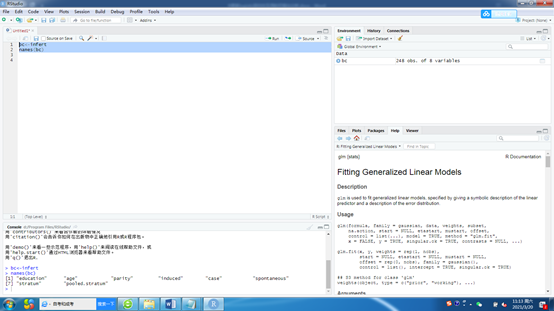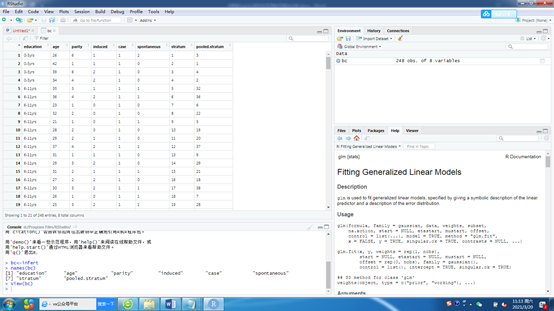数据有8个指标，最后两个是PSM匹配结果，我们不用理他，其余六个为： Education：教育程度，age:年龄，parity产次，induced：人流次数，case：是否不孕，这是结局指标，spontaneous：自然流产次数。 有一些变量是分类变量，我们需要把它转换一下 bc$education<-ifelse(bc$education=="0-5yrs",0,ifelse(bc$education=="6-11yrs",1,2))
bc$spontaneous<-as.factor(bc$spontaneous)
bc$case<-as.factor(bc$case)
bc$induced<-as.factor(bc$induced)
bc$education<-as.factor(bc$education)


建立模型，假如我们想知道流产（包括人流和自然流产）和年龄这两个指标是不是存在交互影响
f1<- glm(case ~ age + education + parity + induced+spontaneous+age*induced*spontaneous,
family = binomial(link = logit), data = bc)
summary(f1)


我们看到自然流产和年龄之间可能存在交互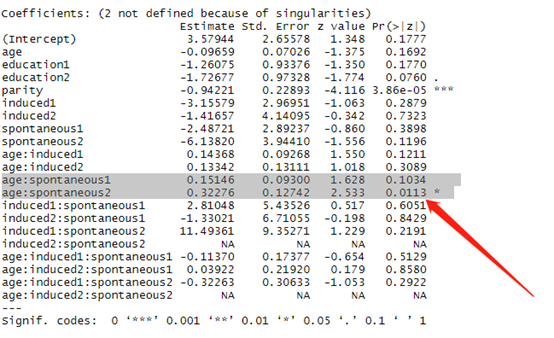我们导入R可视化包visreg
library("visreg")
library("visreg")
plot(visreg(f1,xvar = "age",by="spontaneous",plot=F),xlab="age",ylab="predict",
overlay = T,partial = F,rug=F,,line=list(lty=1:6))
legend("topleft",
c("没有流产","流产1次","流产2次以上"),
lty=c(1,1,1),
col=c("red","green","blue"),
lwd=c(2,1,1),
bty="n")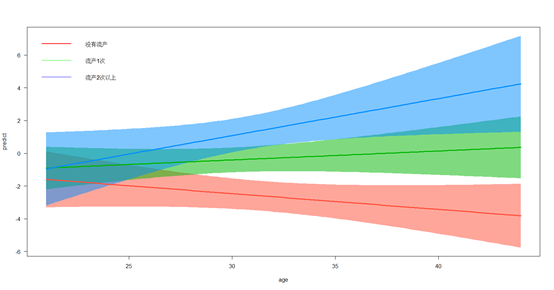由此我们可知，随着年龄增长，自然流产2次以上的患者不孕的概率比没有自然流产的患者明显增高。 更多精彩文章请关注公众号：零基础说科研展开全文可视化
• 多元统计分析 中，交互作用是指某因素作用随其他因素水平的不同而不同，两因素同时存在是的作用不等于两因素单独作用之和(相加交互作用)或之积(相乘交互作用)。通俗来讲就是，当两个或多个因素同时作用于一个结局时...
原文链接：http://tecdat.cn/?p=21892
原文出处：拓端数据部落公众号

引言
多元统计分析 中，交互作用是指某因素作用随其他因素水平的不同而不同，两因素同时存在是的作用不等于两因素单独作用之和(相加交互作用)或之积(相乘交互作用)。通俗来讲就是，当两个或多个因素同时作用于一个结局时，就可能产生交互作用，又称为效应修饰作用(effect modification)。当两个因素同时存在时，所导致的效应(A)不等于它们单独效应相加(B+C)时，则称因素之间存在交互作用。当A=B+C时称不存在交互效应;当A>B+C时称存在正交互作用，又称协同作用(Synergy)。 在一个回归模型中，我们想写的是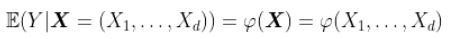当我们限制为线性模型时，我们写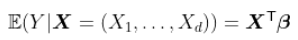或者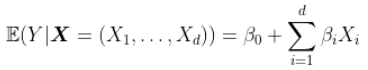但是我们怀疑是否缺少某些因素……比如，我们错过所有可能的交互影响。我们可以交互变量，并假设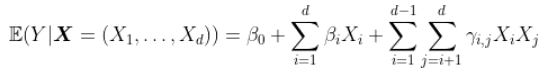可以进一步扩展，达到3阶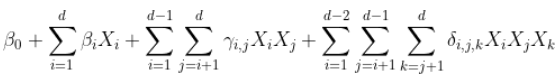甚至更多。
假设我们的变量  在这里是定性的，更确切地说是二元的。

信贷数据
让我们举一个简单的例子，使用信贷数据集。
Credit数据是根据个人的银行贷款信息和申请客户贷款逾期发生情况来预测贷款违约倾向的数据集，数据集包含24个维度的，1000条数据。
该数据集将通过一组属性描述的人员分类为良好或不良信用风险。 数据集将通过一组属性描述的人员分类为良好或不良信用风险。
建立模型
我们读取数据
db=Credit
我们从三个解释变量开始，

reg=glm(Y~X1+X2+X3,data=db,family=binomial)
summary(reg)
没有交互的回归长这样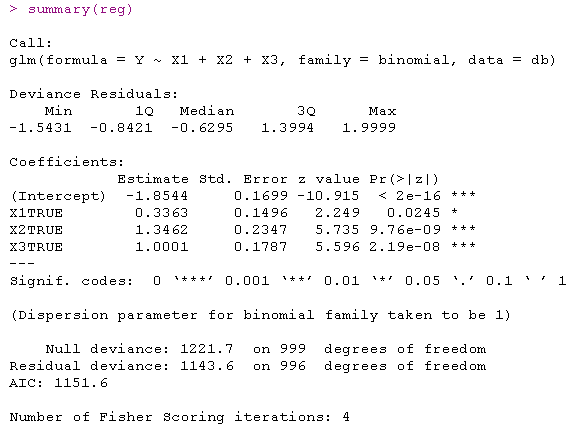这里有几种可能的交互作用（限制为成对的）。进行回归时观察到：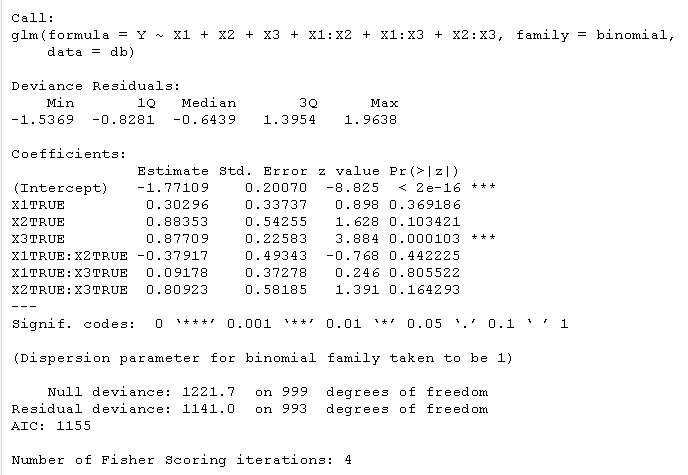交互关系可视化
我们可以画一幅图来可视化交互：我们有三个顶点（我们的三个变量），并且可视化了交互关系

plot(sommetX,sommetY,cex=1,axes=FALSE,xlab="",ylab="",

for(i in 1:nrow(indices)){
segments(sommetX[indices[i,2]],sommetY[indices[i,2]],
text(mean(sommetX[indices[i,2:3]]),mean(sommetY[indices[i,2:3]]),
}

text(sommetX,sommetY,1:k)
这给出了我们的三个变量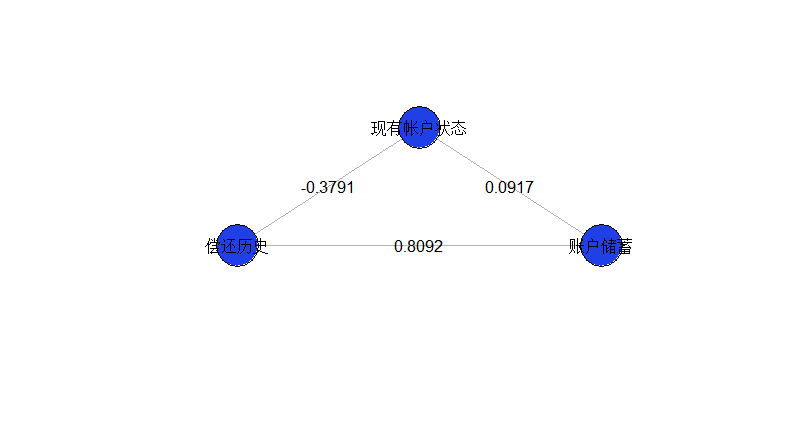这个模型似乎是不完整的，因为我们仅成对地看待变量之间的相互作用。实际上，这是因为（在视觉上）缺少未交互的变量。我们可以根据需要添加它们

reg=glm(Y~X1+X2+X3+X1:X2+X1:X3+X2:X3,data=db,family=binomial)
k=3
theta=pi/2+2*pi*(0:(k-1))/k
plot(X,Y
for(i in 1:nrow(indices)){
segments(X[indices[i,2]],Y[indices[i,2]],
for(i in 1:k){
cercle(c(cos(theta)[i]*1.18,sin(theta)[i]*1.18),.18)
text(cos(theta)[i]*1.35,sin(theta)[i]*1.35,
points(X,Y,cex=6,pch=1)

这里得到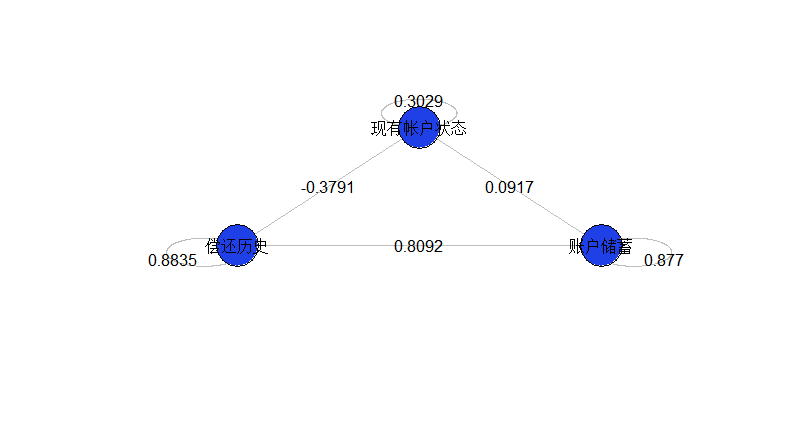如果我们更改变量的“含义”（通过重新编码，通过排列真值和假值），将获得下图

glm(Y~X1+X2+X3+X1:X2+X1:X3+X2:X3,data=dbinv,family=binomial)
plot(sommetX,sommetY,cex=1
for(i in 1:nrow(indices)){
segments(sommetX[indices[i,2]]
for(i in 1:k){
cercle(c(cos(theta)[i]*1.18,sin(theta)[i]*1.18)

points(sommetX,sommetY,cex=6,pch=19)

然后可以将其与上一张图进行比较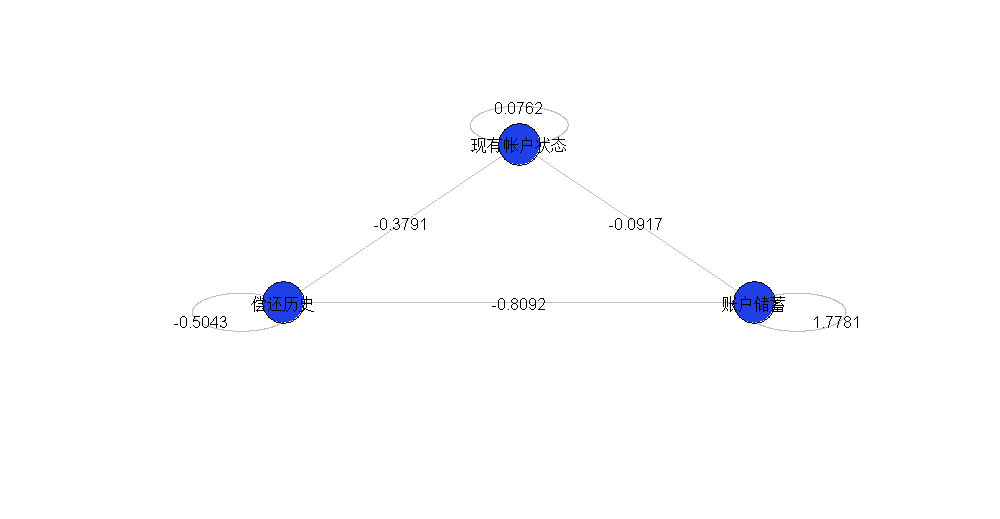使用5个变量，我们增加了可能的交互作用。
然后，我们修改前面的代码

formule="Y~1"
for(i in 1:k) formule=paste(formule,"+X",i,sep="")
for(i in 1:nrow(indices)) formule=paste(formule,"+X",indices[i,2],":X",indices[i,3],sep="")
reg=glm(formule,data=db,family=binomial)
plot(sommetX,sommetY,cex=1
for(i in 1:nrow(indices)){
segments(sommetX[indices[i,2]],sommetY[indices[i,2]],
for(i in 1:k){
cercle(c(cos(theta)[i]*1.18,sin(theta)[i]*1.18)
points(sommetX,sommetY,cex=6

给出了更复杂的图，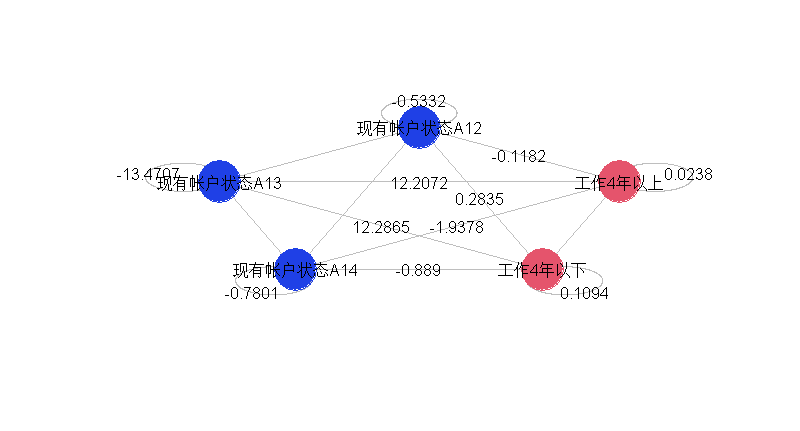我们也可以只采用2个变量，分别取3和4种指标。为第一个提取两个指标变量（其余形式为参考形式），为第二个提取三个指标变量，
formule="Y~1"
for(i in 1:k) formule=paste(formule,"+X",i,sep="")
for(i in 1:nrow(indices)formule=paste(formule,"+X",indices[i,2],":X",indices[i,3],sep="")
reg=glm(formule,data=db,family=binomial)
for(i in 1:nrow(indices){
if(!is.na(coefficients(reg)[1+k+i])){
segments(X[indices[i,2]],Y[indices[i,2]],
}
for(i in 1:k){
cercle(c(cos(theta)[i]*1.18,sin(theta)[i]*1.18),.18)
text(cos(theta)[i]*1.35,sin(theta)[i]*1.35,
}

我们看到，在左边的部分（相同变量的三种指标）和右边的部分不再有可能发生交互作用。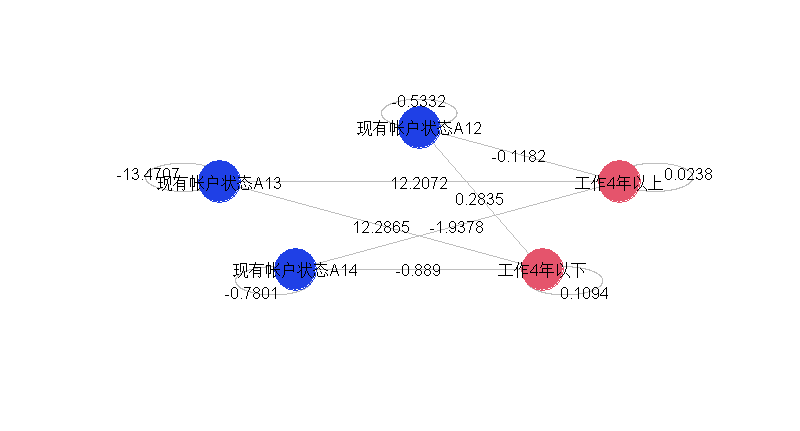我们还可以通过仅可视化显著交互来简化图形。

for(i in 1:nrow(indices)){
if(!is.na(coefficients(reg)[1+k+i])){
if(summary(reg)$coefficients[1+k+i,4]<.1){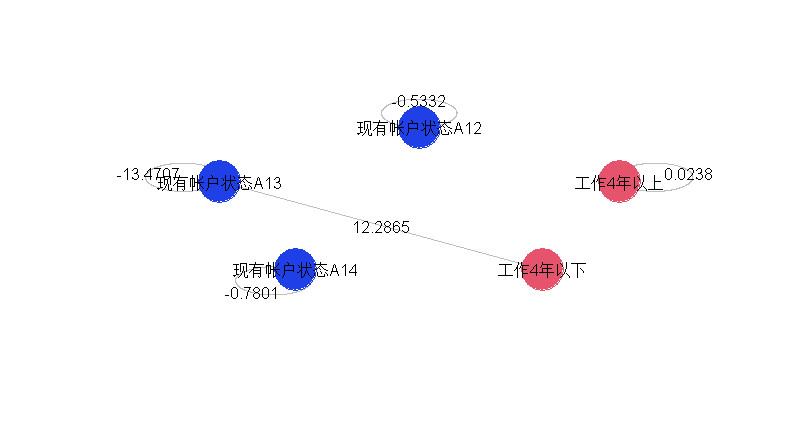在这里，只有一个交互作用是显著的，几乎所有的变量都是显著的。如果我们用5个因子重新建立模型，  for(i in 1:nrow(indices)) formule=paste(formule,"+X",indices[i,2],":X",indices[i,3],sep="") reg=glm(formule,data=db,family=binomial) for(i in 1:nrow(indices){ if(!is.na(coefficients(reg)[1+k+i])){ if(summary(reg)$coefficients[1+k+i,4]<.1){


我们得到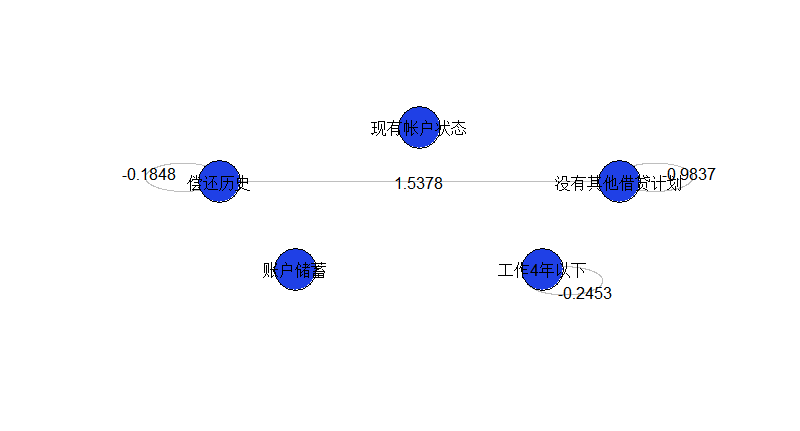最受欢迎的见解
1.R语言多元Logistic逻辑回归 应用案例
2.面板平滑转移回归(PSTR)分析案例实现
3.matlab中的偏最小二乘回归（PLSR）和主成分回归（PCR）
4.R语言泊松Poisson回归模型分析案例
5.R语言回归中的Hosmer-Lemeshow拟合优度检验
6.r语言中对LASSO回归，Ridge岭回归和Elastic Net模型实现
7.在R语言中实现Logistic逻辑回归
8.python用线性回归预测股票价格
9.R语言如何在生存分析与Cox回归中计算IDI，NRI指标
展开全文信用风险 回归 可视化
• Python借助系统执行R语言程序脚本，实现R语言和Python进行程序交互R语言在系统中配置环境变量，保障脚本运行 写一个R语言的程序脚本，放到系统中的任意路径位置，确定cmd的环境变量下R语言的执行命令可以成功...
• R语言中调用Python的模块包，进行调用加载，实现交互和函数共用。
• 如何用r语言的shiny package制作一个交互式页面里这样的tree呢？当改动第金额和人数的数字时，下面的每人平均金额也会改变。提示下应该搜索什么package也可以，我目前用了diagrammeR但是出来的树...css
• 45分钟搞定R语言之数据库交互及统计绘图，压缩包文件，欢迎下载
• 45分钟搞定R语言之数据库交互及统计绘图.pdf
• 本文最近更新地址 本文参考：...Feature是一种文件格式，支持R语言和Python的交互式存储，速度更快。目前支持R语言的data.frame和Python pandas 的DataFrame。Feather收到了Apache arrow 项目的支持python 快速存储 Feature
• 使用C#调用R语言可以选用R.NET，使用时有以下需要注意的地方： 1.安装RDotNet using RDotNet; 2 安装R的版本不能太高，我选用的是R3.4.4 3.配置环境变量 （1）电脑-属性-高级系统设置-高级-环境变量-系统变量-...C#
• 利用R语言进行交互数据可视化 rCharts包 说起R语言交互包，第一个想到的应该就是rCharts包。该包直接在R中生成基于D3的Web界面。 rCharts包的安装 require(devtools) install_github('rCharts', 'ramnathv')...数据可视化...